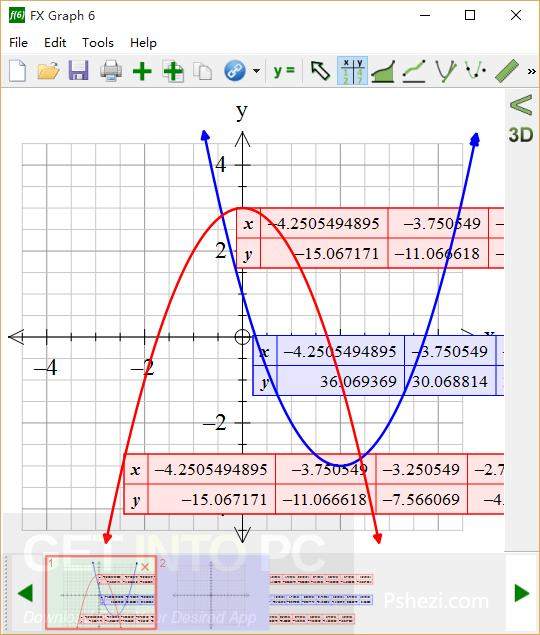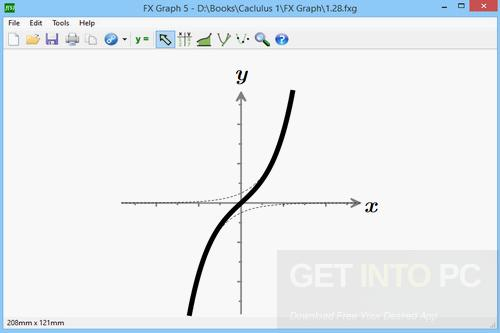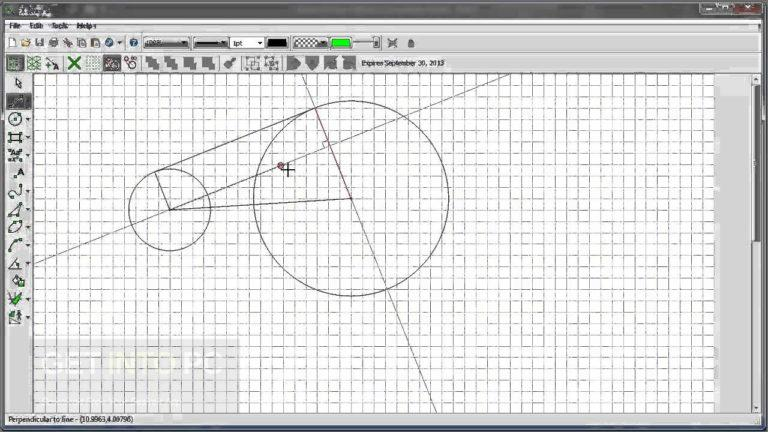Efofex FX MathPack Overview

​Efofex FX MathPack is a tool that has been developed for the Maths teachers. With this complete practical school package you can create graphs, produce statistics and calculate mathematical expressions. Mathematical computation becomes very easy and it can be used for creating test sheets and project graphs. FXdraw, FXEquation, FXStat and FXGraph and each one of them can be used foe making the process of calculating functions and graph creation a simple task. You can also download Wolfram Mathematica 10.4.1.This pack will let you install the components from a single pack and also lets you select the applications which you want to skip. ​Efofex FX MathPack has got an intuitive interface and it will let you draw statistics, distribution curves and graphic representations of curves easily. Complex mathematical functions can also be created which many text editors can’t support. It has got some specialized tools which are FX Draw, FX Equation and FX Graph. FX draw will let you create shapes as well as freeform drawings on the mathematics paper grid. It will also let you generate graphs, illustrations and diagrams. FX Equation will let you create complex mathematical equations which includes integrals, scientific notations, fractions, summation and power or roots. FX Graph can be used for creating graphs and FX Stat is a tool used for generating statistics graphs or tables. All in all ​Efofex FX MathPack is an amazing application which is ideal for teachers and students for exploring advanced math functions. You can also download Wolfram Mathematica 11.0.1.Features of Efofex FX MathPack

Below are some noticeable features which you’ll experience after Efofex FX MathPack free download.

• Impressive tool developed for Maths teachers.
• Complete package for creating graphs, producing statistics and calculating mathematical expressions.
• Mathematical computations becomes very easy.
• ​Efofex FX MathPack has got an intuitive interface.
• Complex mathematical functions can also be created which many text editors can’t support.
• Got 4 impressive tools FX Draw, FX Equation, FX Graph and FX Stat.Efofex FX MathPack Technical Setup Details

• Software Full Name: ​Efofex FX MathPack
• Setup File Name: Efofex_FX_MathPack.zip
• Full Setup Size: 79.8 MB
• Setup Type: Offline Installer / Full Standalone Setup
• Compatibility Architecture: 32 Bit (x86) / 64 Bit (x64)
• Developers: ​Efofex FX